## RS Aggarwal Class 8 Solutions Chapter 1 Rational Numbers Ex 1C

These Solutions are part of RS Aggarwal Solutions Class 8. Here we have given RS Aggarwal Solutions Class 8 Chapter 1 Rational Numbers Ex 1C.

Other Exercises

Question 1.
Solution:
(i) $$\\ \frac { -2 }{ 5 }$$ + $$\\ \frac { 4 }{ 5 }$$
= $$\\ \frac { -2+4 }{ 5 }$$ = $$\\ \frac { 2 }{ 5 }$$Question 2.
Solution:
(i) $$\frac { 3 }{ 4 } +\left( \frac { -3 }{ 5 } \right)$$
$$\frac { 15+\left( -12 \right) }{ 20 } =\frac { 15-12 }{ 20 } =\frac { 3 }{ 20 }$$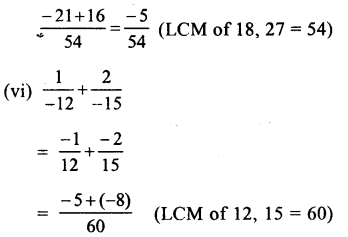Question 3.
Solution:
(i) L.H.S = $$\\ \frac { -12 }{ 5 }$$ + $$\\ \frac { 2 }{ 7 }$$
= $$\\ \frac { -84+10 }{ 35 }$$ = $$\\ \frac { -74 }{ 35 }$$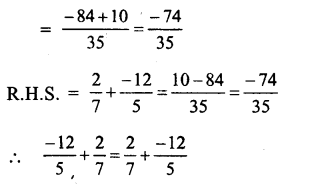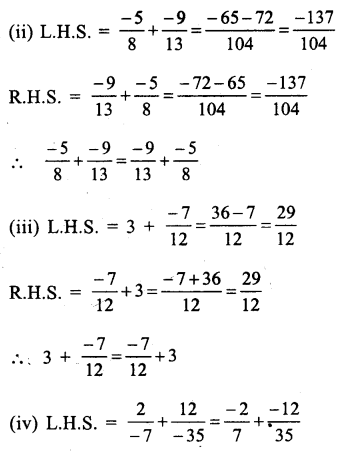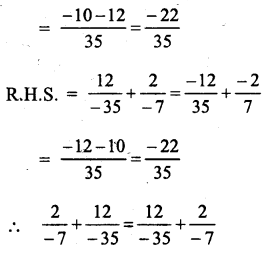Question 4.
Solution: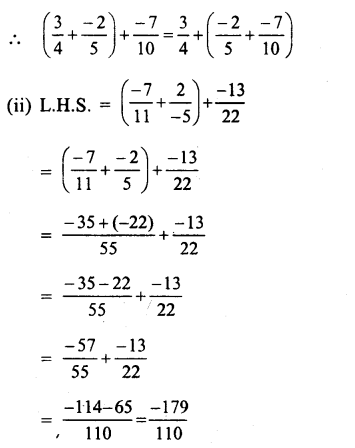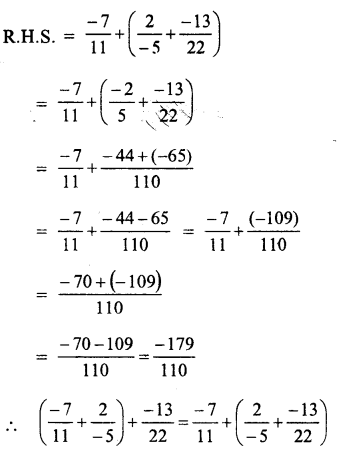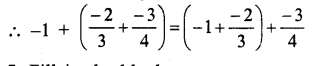Question 5.
Solution: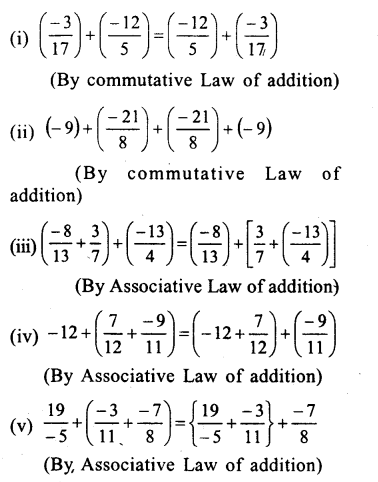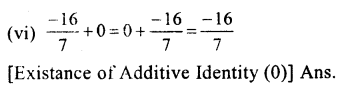Question 6.
Solution:
We know that additive inverse of $$\\ \frac { a }{ b }$$ is $$\\ \frac { -a }{ b }$$ and of $$\\ \frac { -a }{ b }$$ is $$\\ \frac { a }{ b }$$.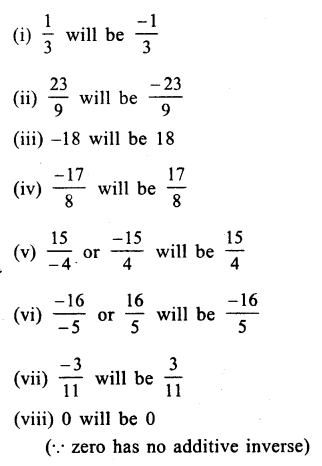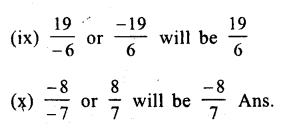Question 7.
Solution:
(i) $$\\ \frac { 3 }{ 4 }$$ from $$\\ \frac { 1 }{ 3 }$$ or $$\\ \frac { 1 }{ 3 }$$ – $$\\ \frac { 3 }{ 4 }$$
= $$\\ \frac { 4-9 }{ 12 }$$ = $$\\ \frac { -5 }{ 12 }$$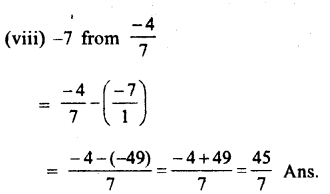Question 8.
Solution: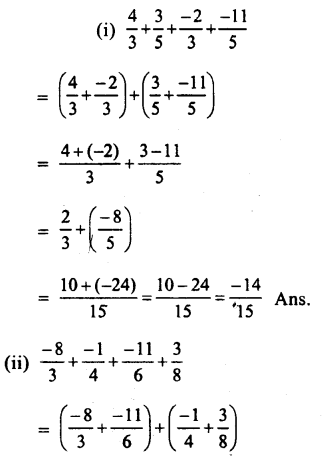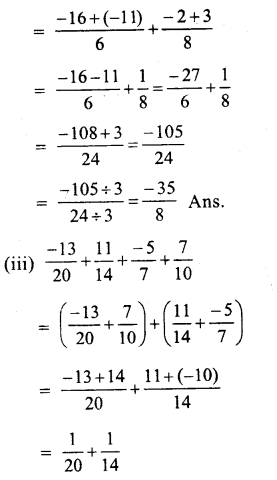Question 9.
Solution:
Sum of two numbers = – 2
one number = $$\\ \frac { -14 }{ 5 }$$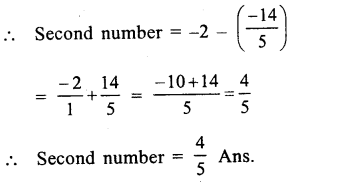Question 10.
Solution:
Sum of two numbers = $$\\ \frac { -1 }{ 2 }$$
One number = $$\\ \frac { 5 }{ 6 }$$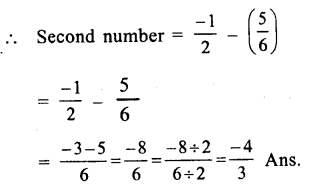Question 11.
Solution:
Sum of two numbers = $$\\ \frac { -3 }{ 2 }$$
one number = $$\\ \frac { -5 }{ 8 }$$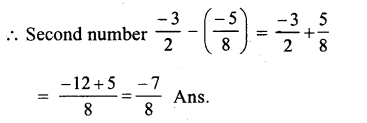Question 12.
Solution:
Sum of two numbers = $$\\ \frac { 5 }{ 7 }$$
One number = – 1
Second number = $$\\ \frac { 5 }{ 7 }$$ – ( – 1)
= $$\\ \frac { 5 }{ 7 }$$ + $$\\ \frac { 1 }{ 1 }$$
$$\\ \frac { 5+7 }{ 7 }$$ = $$\\ \frac { 12 }{ 7 }$$

Question 13.
Solution:
Difference of two numbers = $$\\ \frac { -1 }{ 6 }$$
One number = $$\\ \frac { -2 }{ 3 }$$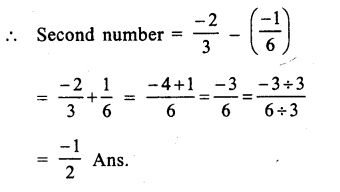Question 14.
Solution:
(i) 0 is the rational number.
(ii) Yes, the difference of two rational numbers is also rational.